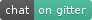Spotlight uses PyTorch to build both deep and shallow recommender models. By providing both a slew of building blocks for loss functions (various pointwise and pairwise ranking losses), representations (shallow factorization representations, deep sequence models), and utilities for fetching (or generating) recommendation datasets, it aims to be a tool for rapid exploration and prototyping of new recommender models.

See the full documentation for details.

Installation¶

conda install -c maciejkula -c pytorch spotlight

Usage¶

Factorization models¶

To fit an explicit feedback model on the MovieLens dataset:

from spotlight.cross_validation import random_train_test_split
from spotlight.datasets.movielens import get_movielens_dataset
from spotlight.evaluation import rmse_score
from spotlight.factorization.explicit import ExplicitFactorizationModel

dataset = get_movielens_dataset(variant='100K')

train, test = random_train_test_split(dataset)

model = ExplicitFactorizationModel(n_iter=1)
model.fit(train)

rmse = rmse_score(model, test)

To fit an implicit ranking model with a BPR pairwise loss on the MovieLens dataset:

from spotlight.cross_validation import random_train_test_split
from spotlight.datasets.movielens import get_movielens_dataset
from spotlight.evaluation import mrr_score
from spotlight.factorization.implicit import ImplicitFactorizationModel

dataset = get_movielens_dataset(variant='100K')

train, test = random_train_test_split(dataset)

model = ImplicitFactorizationModel(n_iter=3,
loss='bpr')
model.fit(train)

mrr = mrr_score(model, test)

Sequential models¶

Recommendations can be seen as a sequence prediction task: given the items a user has interacted with in the past, what will be the next item they will interact with? Spotlight provides a range of models and utilities for fitting next item recommendation models, including

from spotlight.cross_validation import user_based_train_test_split
from spotlight.datasets.synthetic import generate_sequential
from spotlight.evaluation import sequence_mrr_score
from spotlight.sequence.implicit import ImplicitSequenceModel

dataset = generate_sequential(num_users=100,
num_items=1000,
num_interactions=10000,
concentration_parameter=0.01,
order=3)

train, test = user_based_train_test_split(dataset)

train = train.to_sequence()
test = test.to_sequence()

model = ImplicitSequenceModel(n_iter=3,
representation='cnn',
loss='bpr')
model.fit(train)

mrr = sequence_mrr_score(model, test)

Datasets¶

Spotlight offers a slew of popular datasets, including Movielens 100K, 1M, 10M, and 20M. It also incorporates utilities for creating synthetic datasets. For example, generate_sequential generates a Markov-chain-derived interaction dataset, where the next item a user chooses is a function of their previous interactions:

from spotlight.datasets.synthetic import generate_sequential

# Concentration parameter governs how predictable the chain is;
# order determins the order of the Markov chain.
dataset = generate_sequential(num_users=100,
num_items=1000,
num_interactions=10000,
concentration_parameter=0.01,
order=3)

How to cite¶

Please cite Spotlight if it helps your research. You can use the following BibTeX entry:

@misc{kula2017spotlight,
title={Spotlight},
author={Kula, Maciej},
year={2017},
publisher={GitHub},
howpublished={\url{https://github.com/maciejkula/spotlight}},
}

Contributing¶

Spotlight is meant to be extensible: pull requests are welcome. Development progress is tracked on Trello: have a look at the outstanding tickets to get an idea of what would be a useful contribution.

We accept implementations of new recommendation models into the Spotlight model zoo: if you’ve just published a paper describing your new model, or have an implementation of a model from the literature, make a PR!